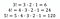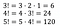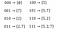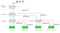# Strategies in Algorithm Design

One very important aspect of problem-solving is devising good strategies. Indeed there are many strategies for algorithm design. This post elaborates more on just a few of them.

The outline of this post is based somewhat on the third chapter of the book Computer Science Distilled . As noted earlier, there are many strategies for algorithm design. A quick run-through of the essential strategies are:

• Iteration
• Recursion
• Brute force
• Backtracking
• Greedy Method (Heuristics)
• Divide and Conquer
• Dynamic Programming
• Branch and Bound
• Two Pointer
• Sliding Window

The focus of this post is to expatiate on the first four: iteration, recursion, brute force and backtracking.

# Algorithm and Strategy

Before moving on, it is important to answer this question: Is an algorithm the same as a strategy?

To answer that question, the definition of these terms might help.

Algorithm: An algorithm is a sequence of computational steps that transform the input to an output. It is a tool for solving a well-specified computational problem. 

Strategy: A strategy is an approach (or a series of approaches) devised to solve a computational problem.

Since both are intended to solve computational problems, how are they related? Simply put:

How are they different?

• A strategy might yield incorrect results, but a correct algorithm will always produce correct results.
• Strategies are invented, algorithms are more or less tested and trusted standards
• Strategies are flexible, but algorithms are rigid i.e. they follow only one set of procedures

Let’s consider, for example, the trivial case of subtracting two numbers where at least one borrow is involved.

An algorithm could be the traditional algorithm for subtraction shown in Figure 1.

However, we could devise other strategies that exploit either the place values of the individual digits that make up the problem or a give and take strategy as shown in Figure 2.

The examples above, though trivial, are just meant to highlight the fact that a difference exists between algorithms and strategies. How so?

Notice that when solving using the traditional algorithm for subtraction, little mental effort was required. We’ve probably been using this strategy since elementary school for basic subtractions when a borrow is involved. However, the other strategies depicted in Figure 2 required us to think. Therein lies the difference — thinking.

One needs to thoroughly analyse the problem statement and make sure he understands it inside out. This is important before diving into the code or implementation.

The four basic strategies for performing computational tasks now follows.

# 1. Iteration

Iteration can be achieved using loops or recursion (more on this later). The basic loop constructs are:

• The for loop
• The for-each loop
• The while loop
• The do-while loop

Some functions based on the above loop constructs are the filter, findAny, and reduce functions.

# 2. Recursion

This base case ensures that the solution converges otherwise an infinite recursion occurs which in turn leads to a Stack Overflow.

Recursion is intuitive because each new method call works on clones of the original problem leading to a final result (if it converges).

# Problem 1

Find the factorial of any positive integer n.

## Analysis

Mathematically, the factorial of a positive number, n, is the product of all the consecutive numbers from 1 to n. Thus, the formula is:

For example, if n = 3, 4, and 5, then the result in Figure 4 is expected.Figure 4: Examples of n! when n = 3, 4, and 5

## Using Iteration

This solution can be achieved in a variety of ways with iteration. Code snippet #1 makes use of the for loop, while loop and the reduce function. The loop is repeated n times. Thus, the time complexity for the factorial function is O(n).

Complexity: O(n)

## Using Recursion

To better understand how recursion would work for this problem, insight is needed. Notice that factorial function in Figure 3 is simply calling itself with smaller values of n. Thus, when the result of smaller subproblems is known, we can easily compute the result of other higher problems. This is highlighted in Figure 5.

Again, since at most n method calls are made during recursion, the time complexity is O(n).Figure 5: Examples of n! as a function of solutions to previous subproblems

Complexity: O(n)

# Problem 2

Generate all subsets of a set of n elements.

## Analysis

The problem can be rephrased as find all combinations of a set of n elements. For example, the subsets of numbers 5, 2, and 7 are as shown in Figure 6.

There are different ways to solve this problem. However, one strategy is to exploit the bit manipulation of integers via the power set. How so?

## Using Iteration

As shown in Figure 8, since we have three items, the number of subsets is the same as 2³ (two to the third degree) which is 8. Thus, we only need to consider the binary representation of the numbers from 0 to 2³ - 1 and for each set bit, create a subset from it (Figure 7). A bit is set if its value is 1.

The time complexity for this solution is O(2^n) since iteration is done 2³ times where n = 3.Figure 7: Binary representation of the subsets of the set {5, 2, 7}

Complexity: O(2^n)

## Using Recursion

Again, another strategy to solve this problem is via recursion. Figure 8 is an aid to understanding this solution.

Notice there is a list called currentSubset as well as a subsets list. The currentSubset is meant to be a dynamic list with lots of insert-remove operations. The subsets list is a two-dimensional list to store, well, the subsets of the set.

There are four basic steps needed in this implementation (assuming i is our current index value, and subset(i) is our recursive function):

• call subset(i+1)
• add an element at index i to the current subset
• call subset(i+1) again
• remove (pop) most recently added element from the current subset

These four steps are just about highlighted with green, brown, blue, pink arrows respectively.Figure 8: An overview of the method calls on the subset function when i starts from zero

The idea is simple. We consider each element (at current index i) of the set as either:

1. not part of the current subset or
2. part of the current subset

during the method calls. That means the second recursive call alone is made with the element at index i as part of the current subset. Notice also the binary nature of this assumption.

The recursion starts at an index of zero and converges when the index is equal to the size of elements in the set. This base condition is highlighted in green in Figure 8. Every single time the base condition is reached, a clone of the current subset is inserted into the 2D subsets list.

After the second recursive call, the recently added item is popped from the current subset. Code snippet #4 shows the implementation in Java. The complexity is also O(2^n) because 2^n - 1 method calls are made (shown in the next section).

Complexity: O(2^n)

## Iteration or Recursion?

It depends. If elegance and simplicity are required, then recursion is the way to go. However, in resource-limited situations where speed and performance are prioritised, iteration would be better suited.

# 3. Brute force

By using this approach, if a solution exists, it is guaranteed the solution will be found. However, the time required to get such a solution might be impractical. Thus, brute force strategies are generally computationally expensive even for small inputs.

Problem 2 above is such an example.

# 4. Backtracking

Thus, backtracking adds “intelligence” to the brute force strategy. Why the need for intelligence? Well, if you know a partial solution leads to a dead-end (or an eventual incorrect solution), then there is no need going further with the partial solution. It is simply discarded.

# Problem 3

Find the subsets of a set of n unique elements whose sum add up to K.

## Analysis

The problem statement is a variant of Problem 2. Some examples for different values of K (1≤K≤15) are shown below.

## Using Brute Force

We simply modify the recursive solution to Problem 2 such that when we reach the base case, we check to see if the sum of the current subset equals K. If this condition is true, then we add this solution to the 2D subsets list.

When the method in Code snippet #5 is called with our sample input S={5, 2, 7}, it yields the following result:

`Subsets of [5, 2, 7] with sum 0: [[]]. Method calls: 15Subsets of [5, 2, 7] with sum 1: []. Method calls: 15Subsets of [5, 2, 7] with sum 2: []. Method calls: 15...Subsets of [5, 2, 7] with sum 14: [[5, 2, 7]]. Method calls: 15Subsets of [5, 2, 7] with sum 15: []. Method calls: 15`

Consider also the first 10 unique elements of the Fibonacci series S = {0, 1, 2, 3, 5, 8, 13, 21, 34, 55}, the result in this case is:

`Subsets of [0, 1, 2, 3, 5, 8, 13, 21, 34, 55] with sum 0: [[], ]. Method calls: 2047Subsets of [0, 1, 2, 3, 5, 8, 13, 21, 34, 55] with sum 1: [, [0, 1]]. Method calls: 2047Subsets of [0, 1, 2, 3, 5, 8, 13, 21, 34, 55] with sum 2: [, [0, 2]]. Method calls: 2047...Subsets of [0, 1, 2, 3, 5, 8, 13, 21, 34, 55] with sum 14: [[1, 13], [1, 5, 8], [1, 2, 3, 8], [0, 1, 13], [0, 1, 5, 8], [0, 1, 2, 3, 8]]. Method calls: 2047Subsets of [0, 1, 2, 3, 5, 8, 13, 21, 34, 55] with sum 15: [[2, 13], [2, 5, 8], [0, 2, 13], [0, 2, 5, 8]]. Method calls: 2047`

In both examples and for all possible values of K, the method _subsetSum is called 2^n - 1) times. This is quite inefficient because some subsets can never yield a solution when K is minimal.

Complexity: O(2^n)

## Using Backtracking

In this case, we improve upon the brute force strategy earlier devised. Common sense dictates that when the current sum of the dynamic subset exceeds our target sum K, then there is no point proceeding further. Thus the process backtracks and moves on to other viable solutions. That is one of many ways this solution can be optimized.

Again, when S={5, 2, 7}, the optimized method yields the result:

`Subsets of [5, 2, 7] with sum 0: [[]]. Method calls: 7Subsets of [5, 2, 7] with sum 1: []. Method calls: 7Subsets of [5, 2, 7] with sum 2: []. Method calls: 9...Subsets of [5, 2, 7] with sum 14: [[5, 2, 7]]. Method calls: 15Subsets of [5, 2, 7] with sum 15: []. Method calls: 15`

Also, when S = {0, 1, 2, 3, 5, 8, 13, 21, 34, 55}, the result is:

`Subsets of [0, 1, 2, 3, 5, 8, 13, 21, 34, 55] with sum 0: [[], ]. Method calls: 39Subsets of [0, 1, 2, 3, 5, 8, 13, 21, 34, 55] with sum 1: [, [0, 1]]. Method calls: 71Subsets of [0, 1, 2, 3, 5, 8, 13, 21, 34, 55] with sum 2: [, [0, 2]]. Method calls: 99...Subsets of [0, 1, 2, 3, 5, 8, 13, 21, 34, 55] with sum 14: [[1, 13], [1, 5, 8], [1, 2, 3, 8], [0, 1, 13], [0, 1, 5, 8], [0, 1, 2, 3, 8]]. Method calls: 567Subsets of [0, 1, 2, 3, 5, 8, 13, 21, 34, 55] with sum 15: [[2, 13], [2, 5, 8], [0, 2, 13], [0, 2, 5, 8]]. Method calls: 595`

Notice that in both cases above, by eliminating subsets that are not feasible, the number of method calls reduced significantly. For example, for the second test case, when K = 0 and K = 15, the method calls reduced by a factor of 52.49 and 3.44 respectively.

Still, the time complexity of the backtracking approach is the same as the brute force approach. However, with backtracking, it is significantly faster.

Complexity: O(2^n)

# Conclusion

Indeed, there are many strategies one can use to design algorithms needed to solve computational problems. The choice lies with you. When making the decision, remember to consider the following:

• Available resources (CPU cycles, memory etc.)
• Problem size
• Time complexity

When this analysis has been done properly, you’ll find it easier to create optimal solutions.

# References

1. F. F. Wladston, Computer Science Distilled: Learn the Art of Solving Computational Problems. Code Energy LLC (2017)
2. T. H. Cormen, C. E. Leiserson, R. L. Rivest, and C. Stein, Introduction to Algorithms. MIT Press, Cambridge, MA, 3rd edition (2009)

Written by

## More From Medium

#### More from The Startup

Mar 24 · 5 min read

#### More from The Startup

Mar 29 · 9 min read

#### More from The Startup

Mar 25 · 8 min read

#### 1.7K

Welcome to a place where words matter. On Medium, smart voices and original ideas take center stage - with no ads in sight. Watch
Follow all the topics you care about, and we’ll deliver the best stories for you to your homepage and inbox. Explore
Get unlimited access to the best stories on Medium — and support writers while you’re at it. Just \$5/month. Upgrade